UnboundEd Mathematics Guide

# Operations & Algebraic Thinking: Unbound A Guide to Grade 2 Mathematics Standards

?
What's in a Content Guide and how do I use it?
Get answers to all your Content Guide questions, including what's in each part and how they can be used in your role at your school
View FAQs

2.OA.A | Represent and solve problems involving addition and subtraction.

2.OA.B | Add and subtract within 20.

2.MD.D | Represent and interpret data.

Welcome to the UnboundEd Mathematics Guide series! These guides are designed to explain what new, high standards for mathematics say about what students should learn in each grade, and what they mean for curriculum and instruction. This guide, the first for Grade 2, includes three parts. The first part gives a “tour” of the standards for the first two clusters of Operations & Algebraic Thinking (2.OA.A and 2.OA.B) using freely available online resources that you can use or adapt for your class. The second part shows how Operations & Algebraic Thinking relate to representing and interpreting data in Grade 2 (2.MD.D). And the third part explains where the skills and understandings within 2.OA and 2.OA.B are situated in the progression of learning in Grades K-2. Throughout all of our guides, we include a large number of sample math problems. We strongly suggest tackling these problems yourself to help best understand the methods and strategies we’re covering, and the potential challenges your students might face.

# Part 1: What do these standards say?

Much of students’ early learning in mathematics revolves around the operations of addition, subtraction, multiplication and division. But as we know from experience, being able to calculate an answer isn’t enough; students need to have a complete understanding of each of these operations in order to become flexible problem solvers and achieve success in later grades. The standards in the Operations & Algebraic Thinking (OA) domain, which runs from Kindergarten through Grade 5, are designed to meet this need. The OA standards specify the meanings and properties of each operation, fluencies that students should achieve at each grade level, and the types of problems they should be able to solve. In Grade 2, where the emphasis is on addition and subtraction, the standards focus on using mental strategies to add and subtract within 20 and using addition and subtraction to problem solve within 100. (2.OA.A.1, 2.OA.B.2)

The other cluster discussed in this guide comes from the Measurement & Data (MD) domain. This is a supporting cluster, and the standards in this cluster can help students deepen their understanding of the standards in the OA domain. We will talk about these standards in detail in Part 2.

It’s important to note that the clusters, and the standards within the clusters, are not necessarily sequenced in the order in which they have to be taught. (Standards are only a set of expectations of what students should know and be able to do by the end of each year; they don’t prescribe an exact sequence or curriculum.) So planning your instruction sequence carefully can ensure your students continue to build on previous understandings. As we go, think about the connections you see between standards, and how you can use these connections to help students build on their previous understandings.

Also, manipulatives are frequently a large part of the tasks and lessons that follow. They are most effective when connected to written methods, rather than taught as a method for computing or solving unto themselves. Used this way, manipulatives can be an important way to build conceptual understanding of numbers and operations.2

Throughout the guide, we’ll look at examples of tasks and lessons that focus on students’ abilities to make sense of problems and persevere in solving them (MP.1). As students are exposed to varied contexts and problem types, they will need to think carefully to understand each problem and develop an appropriate solution method.

Let’s begin our discussion of the OA standards in Grade 2 by taking a deep look at the second cluster, 2.OA.B. As we dig into this cluster, we will also examine some of the Grade 1 standards and a few of the Kindergarten standards. This is necessary because the strategies required for addition and subtraction in Grade 2 are defined in the Grade 1 standards. In particular, the mental strategies mentioned in 2.OA.B.2 are described in 1.OA.C.6. And the strategies in 1.OA.C.6 are directly supported by important understandings in the Kindergarten standards.

 K.CC.A.2 “Count forward beginning from a given number...” K.OA.A.3 “Decompose numbers less than or equal to 10 into pairs in more than one way…” K.OA.A.4 “For any number from 1 to 9, find the number that makes 10 when added to the given number…” 1.OA.C.6 “Add and subtract within 20, demonstrating fluency for addition and subtraction within 10. Use strategies such as counting on; making ten...decomposing a number leading to a ten...using the relationship between addition and subtraction...and creating equivalent but easier or known sums...” 2.OA.B.2 “Fluently add and subtract within 20 using mental strategies.” (See standard 1.OA.C.6 for a list of mental strategies.)

The progression for addition and subtraction is thoughtfully articulated in the language of the standards. The Grade 1 standards, in particular, shed a lot of light on the culminating expectations set by the Grade 2 standards. In Grade 2 we can best support students along the progression by helping them deepen their understanding of addition and subtraction and by increasing their flexibility with strategies. But we want to be sure to do all of this without sacrificing their conceptual understanding.

Let’s begin by reading the standard in cluster 2.OA.B, and then we’ll think through what it means and how it looks in practice.

2.OA.B | Add and subtract within 20.

 2.OA.B.2 Fluently add and subtract within 20 using mental strategies. By end of Grade 2, know from memory all sums of two one-digit numbers.* *See standard 1.OA.6 for a list of mental strategies.

## Methods for single-digit addition and subtraction

The Grade 1 standard, 1.OA.A.6, states that students should use strategies such as “counting on,” “making ten,” “decomposing a number leading to a ten,” and “creating equivalent but easier or known sums” as a means of achieving fluency. These strategies are part of a hierarchy of computation methods for single-digit addition and subtraction. These methods can be thought of in terms of levels.

• Level 1: Counting all
• Level 2: Counting on
• Level 3: Convert to an easier problem

It’s important to understand these levels, and the appropriate prerequisites, so that we can support students with using increasingly advanced methods. Students primarily work with the Level 1 method in Kindergarten, then move on to the more efficient and widely applicable strategies of Levels 2 and 3 in Grade 1. Our goal in Grade 2 is to build students’ fluency and flexibility with Level 2 and 3 strategies so that they can perform addition and subtraction computations within 20 mentally. (2.OA.B.2) Let’s take a closer look at what these levels mean and how they’re tied to the standards.

### Level 1: Counting all

Using the Level 1 method (i.e., direct modeling by counting all or taking away), students use manipulatives or drawings to model putting together each addend (a number that is added to another number) and then counting the total (in the case of addition). In the case of subtraction, students model the total, then take away an addend, and then count the remaining objects to determine the result. The diagram below illustrates count-all and take-away (and count-remaining) strategies.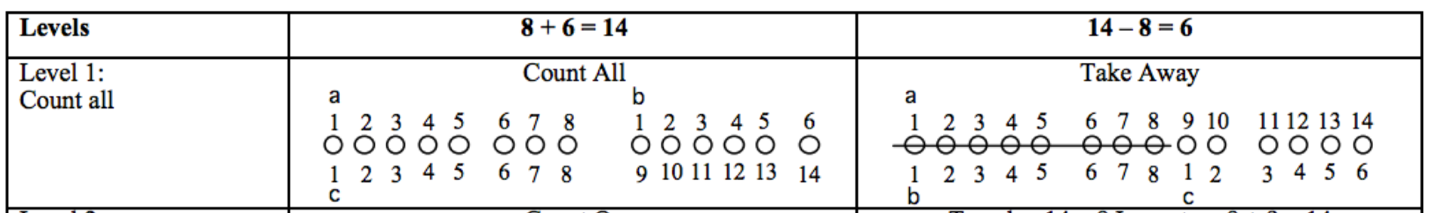This strategy is tied closely to the foundational work with counting that students do in Kindergarten. While this is not one of the mental strategies students should be using in Grade 2, understanding this strategy will help us to coherently introduce new strategies and support students that may not be on grade level.

### Level 2: Counting on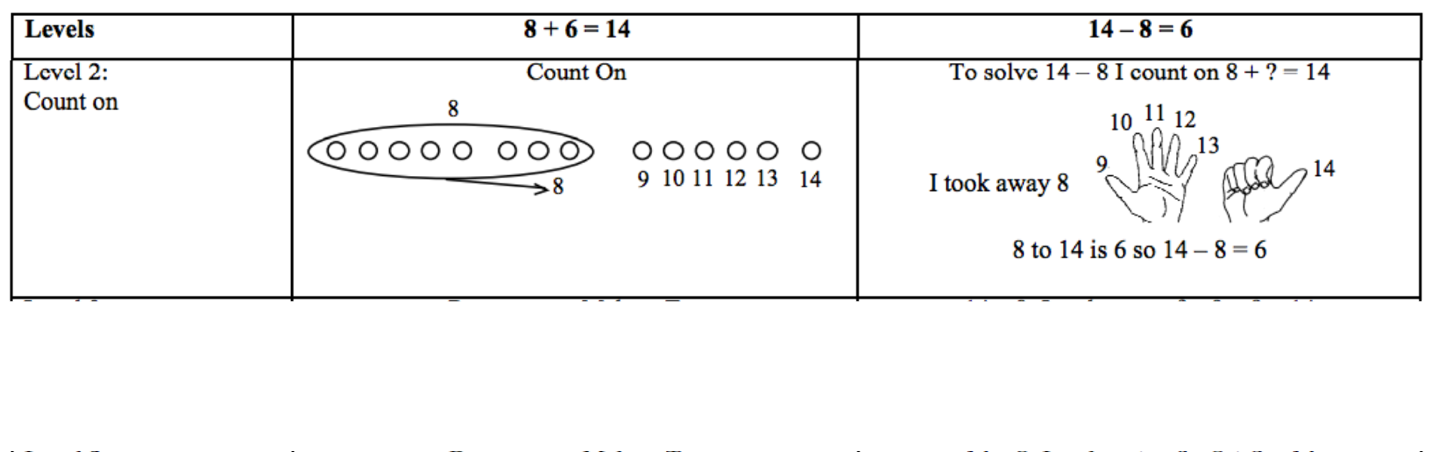This thinking, of course, can be applied to word problems as well. The example below is an “Addend Unknown” problem (more on what that means in a moment) and could be solved by counting on:

#### EngageNY Grade 2, Module 1, Lesson 7: Homework

Robert has 16 cups. Some are red. Nine are blue. How many cups are red?

Using counting on, students would start with 9, count to 16 (e.g., “niiiiine, ten, eleven, twelve, thirteen, fourteen, fifteen, sixteen) while tracking the count of numbers on their hands, to determine the number of red cups to be 7.

Level 3 methods (i.e., convert to an easier problem) are the most sophisticated strategies for adding and subtracting single-digit numbers, and also the most efficient. These strategies include “making 10,” “decomposing a number leading to a 10 and creating equivalent but easier or known sums (e.g., doubling plus or minus 1, or plus or minus 2) ” and are listed as strategies that students should do mentally in Grade 2. Extensive work with Level 3 methods is important in Grade 2, as these make up most of the strategies referenced from Grade 1. (1.OA.C.6). Remember: our goal in Grade 2 is to build students’ fluency and flexibility with Level 2 and 3 strategies so that they can perform addition and subtraction computations within 20 mentally. (2.OA.B.2) In the example below, students decompose a number leading to a ten in order to subtract.

#### EngageNY Grade 2, Module 1, Lesson 7: Problem Set

Shane has 12 pencils. He gives some pencils to his friends. Now, he has 7 left. How many pencils did he give away?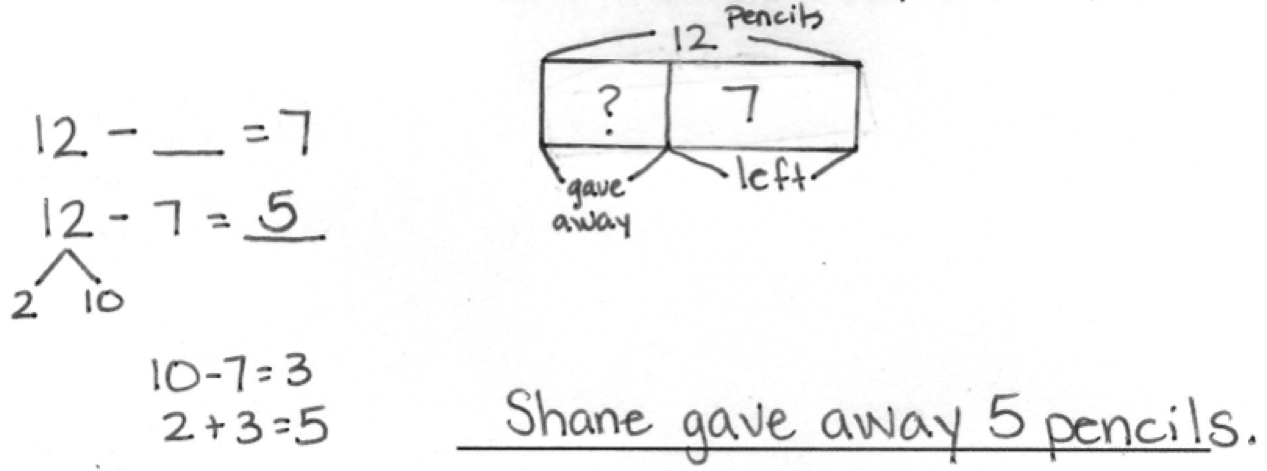Extensive work in K-1 supports knowing decomposition of numbers within 20. (K.OA.A.3, K.OA.A.4, 1.NBT.B.2) Since students are fluent with pairs of addends of 10, subtracting 7 from 10 is easier than subtracting 7 from 12.

Our goal in Grade 2 is to give students enough opportunity and support to engage with these computation strategies so that they can do them mentally. These strategies support fluency with single-digit sums (and related subtraction), but they also support addition and subtraction strategies with larger numbers. For example, imagine if the word problem above was changed to: Robert has 40 cups. Some are red. Eighteen are blue. How many cups are red? Students might solve this problem by counting on with ones and tens (e.g., 18… 28, 38, 39, 40), since students learned to mentally find 10 more or 10 less than any number in Grade 1. (1.NBT.C.5 )That said, when adding and subtracting two- and three-digit numbers, Level 3 methods and strategies based on place value (which are supported by Level 3 methods) become much more efficient than counting on. For example, using the example of Robert and his cups, students might quickly add 2 to make 20 (not make a 10, but make the next 10) and then add 20 to get 40. Then they would add 2 + 20 to determine there are 22 red cups. When we discuss problem solving within 100, we will see more examples of how Level 3 methods support addition and subtraction with two- and three-digit numbers.

Students will apply and often combine these properties of addition to support making an easier problem; for this reason they are sometimes referred to as a single property (“any order, any grouping”) at this grade level. This leads to an important note about properties at this grade level: The Standards dont require that students know the formal names of the properties, as this distracts from the conceptual understanding of these properties. The focus is on applying properties for the purposes of solving problems.

In addition to applying the properties of addition, understanding the relationship between addition and subtraction is described as a mental strategy. Specifically, students come to understand that a subtraction problem can be thought of as an unknown addend problem. For example, in the problem above about Shane’s pencils, students could have solved the problem 12 - ? = 5 by thinking 5 + ? = 12.

## Fluency activities

There are many activities that can build fluency from a conceptual foundation; a step in this direction is often accomplished by paying attention to the numbers we use in our examples. Rather than asking students to recite the answers to a more or less “random” collection of addition and subtraction facts, we can choose facts that illuminate certain strategically advantageous structures. The example below focuses on the relationship between pairs that add to 10:

#### EngageNY Grade 2, Module 1, Lesson 6: Fluency

Take from Ten (2 minutes)

Note: This activity develops the automaticity necessary to subtract fluently from the ten when using the take from ten strategy in Lessons 6, 7, and 8.

T: When I say 10 – 9, you say 10 – 9 = 1. Ready? 10 – 9.

S: 10 – 9 = 1.

T: 10 – 5.

S: 10 – 5 = 5.

Continue with the following sequence: 10 – 2, 10 – 4, 10 – 6, 10 – 7, 10 – 3, and 10 – 8.

T: When I say 1, you say 9. Ready? 1.

S: 9.

T: 2.

S: 8.

Continue with the following sequence: 5, 0, 4, 7, 3, 8, 6, and 10.

This example supports mental strategies by highlighting the different decompositions of 10, which are advantageous in determining other sums and differences within 20.

So far we’ve looked at some of the Grade 2 fluencies plus the associated computation methods, and important understandings regarding the properties of addition and the relationship between addition and subtraction. Now let’s look more closely at how students apply these methods and understandings as strategies for solving addition and subtraction word problems. We’ll also examine how place value understandings play a role. Let’s begin by reading the standard in cluster 2.OA.A.

2.OA.A | Represent and solve problems involving addition and subtraction.

 2.OA.A.1 Use addition and subtraction within 100 to solve one- and two-step word problems involving situations of adding to, taking from, putting together, taking apart, and comparing, with unknowns in all positions, e.g., by using drawings and equations with a symbol for the unknown number to represent the problem.* *See Glossary, Table 1.

## Problem-solving with addition and subtraction

Extensive work with problem-solving is really important in Grades K-2. Using addition and subtraction to solve real-world problems helps students develop meaning for addition and subtraction, fosters growth with computation strategies, and helps them develop fluency with addition and subtraction facts. The types of word problems referred to in 2.OA.A.1 are further delineated into 12 subtypes as demonstrated in Table 1 of the standards.4 And the table below, adapted from the Standards, highlights the specific subtypes that students should master at each grade level.5 By the end of Grade 2, students should master all of the problem types in the table. It will be helpful to spend some time digging into the different problem situations shown in the table, as we will refer to them extensively throughout the rest of this discussion.

Many of us have learned that solving word problems involves finding “key words,”— words like “more” and “total” tell us to add, while words like “fewer” and “less” tell us to subtract. But what about a problem like this:

Lucy has six fewer apples than Julie. Lucy has eight apples. How many apples does Julie have?

The “key word” in this problem, “fewer,” actually hints at the wrong operation; subtracting will not result in the correct answer. A better way to help students with problem-solving is to help them think situationally about the varied contexts. Using word problems to create meaning for operations helps students to better understand how to apply operations.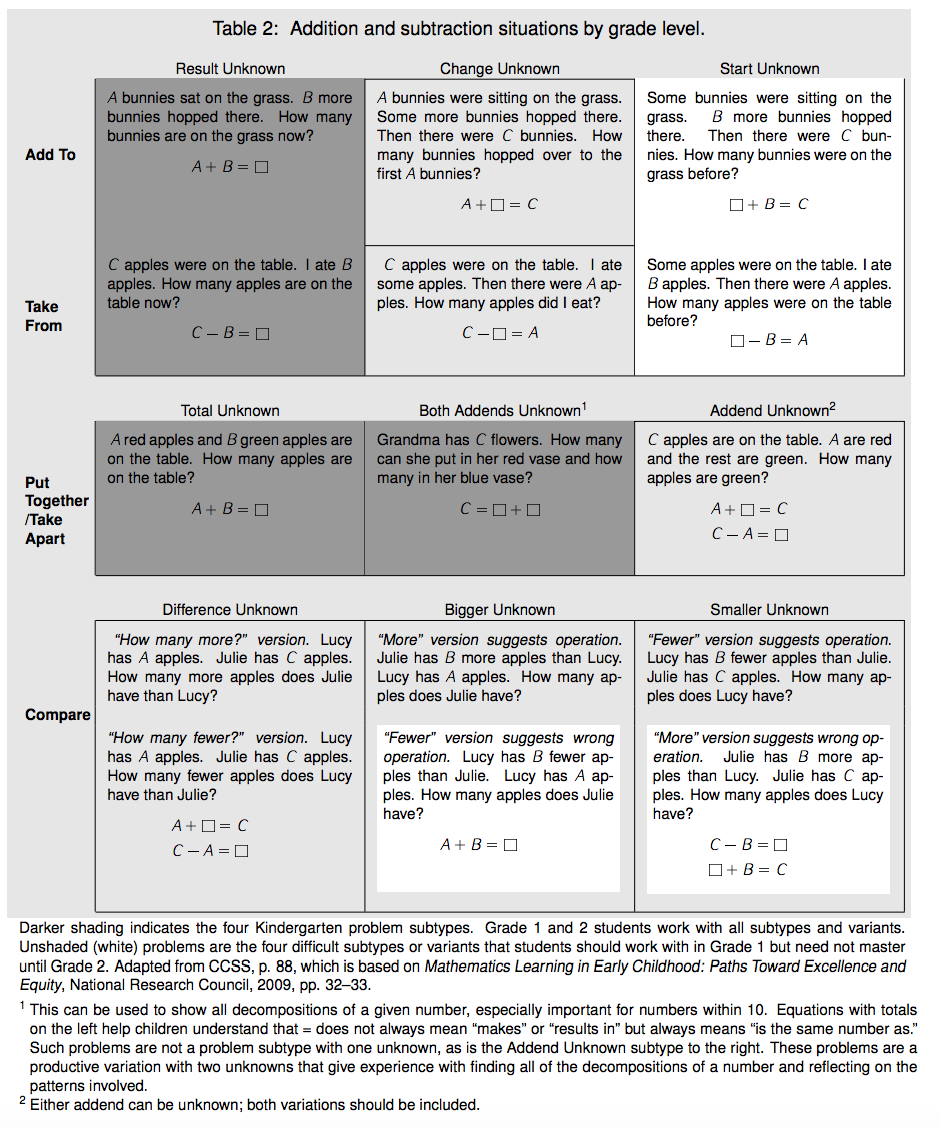Students engage with these types of problems beginning in Kindergarten. In Grade 2, they extend previous work from Kindergarten and Grade 1 in three ways:6 ibid. p. 9. ibid. p. 9. ibid. p. 9.

• Students master all 12 subtypes shown in the table above.
• Students extend problem-solving to whole numbers within 100.
• Students solve two-step problems.

### “Add to with start unknown”

This is one of the more difficult subtypes that students engage with in Grade 2. In the example below, students are trying to determine how much money there was to begin with:

#### Grade 2, Module 4, Lesson 2: Application

Susan has 57 cents in her piggy bank. If she just put in 30 cents today, how much did she have yesterday?

Note: This add to with start unknown problem gives students a chance to apply their new learning. It also provides an opportunity to work through a common mistake; many students will add and give the answer 87 cents. Encourage students to draw a tape diagram to show what is known. This will help them identify the whole and one part, guiding them to subtract to find the missing part.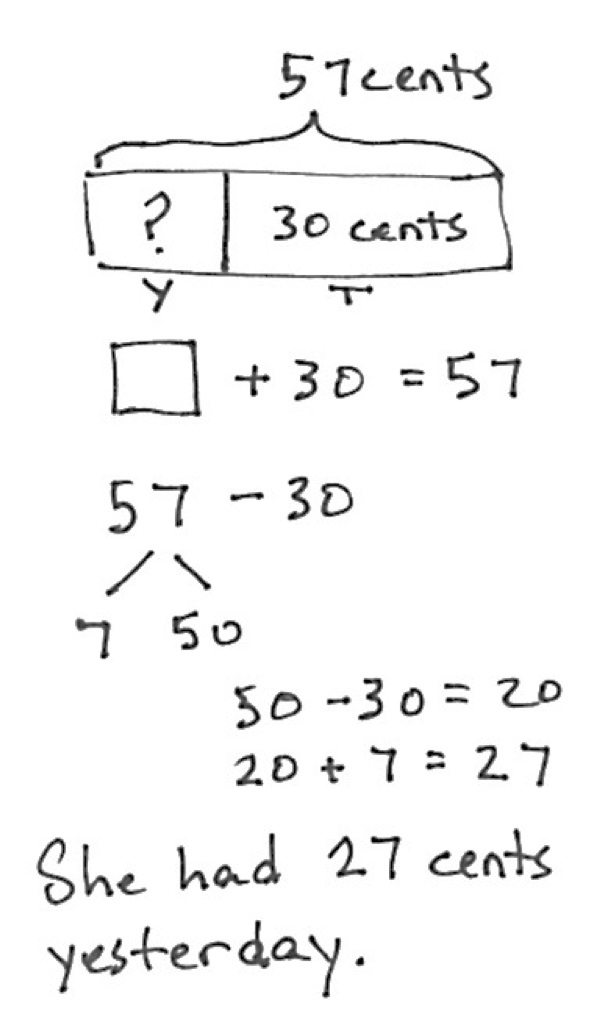HideShow

This kind of problem is challenging because it is difficult to determine what the unknown is; is it an addend or the total? Using diagrams, such as the tape diagram shown, to represent the knowns will help students identify the unknowns. Computationally, this student demonstrates making an easier problem (Level 3) using place value strategies by decomposing 57 into tens and ones and then subtracting 3 tens from 5 tens. Understanding that a two-digit number is composed of tens and ones is a first-grade understanding, (1.NBT.B.2) as is subtracting multiples of ten from two-digit numbers. (1.NBT.C.6)

### “Take from with start unknown”

This is another of the more difficult subtypes that students engage with in Grade 2. Similar to “add to with start unknown” problems, it can be challenging to determine what the unknown is. In the example below, students are trying to determine how many cupcakes there were to begin with.

#### Grade 2, Module 4, Lesson 8: Application

At the school fair, 29 cupcakes were sold, and 19 were left over. How many cupcakes were brought to the fair?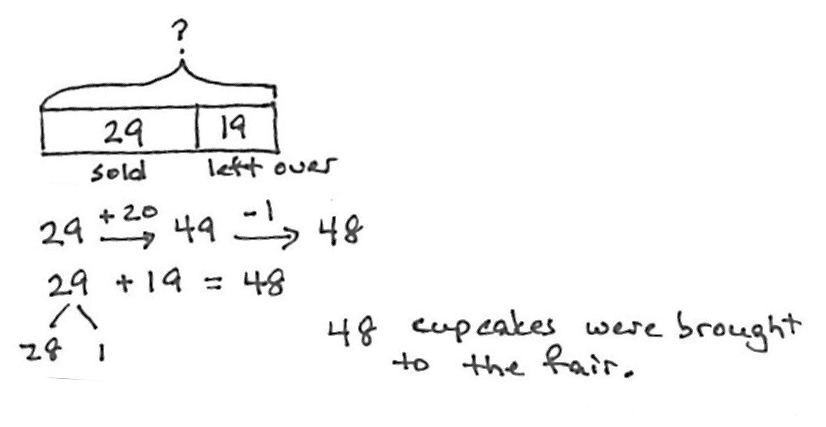Note: This problem is intended for guided practice to help students gain familiarity with the take from with start unknown problem type. The language of these problem types can be confusing to students. Guide students to see that when both parts are known, we add to find the total.

HideShow

As we’ve discussed, in Grade 2 our focus should be on ensuring students can apply Level 3 methods—in which they convert to an easier problem—when solving any of the subtypes described in the table above. Asking students to show or explain their thinking in problems will help us understand what levels our students are using, and how we can support them in flexibly employing the advanced Level 3 strategies necessary for Grade 2 work. In the example above, both strategies demonstrate making an easier problem. In the first strategy, a student adds 1 to 19 to make a multiple of 10: adding 20 to 29 is easier than adding 19 to 29. Then the student remembers to subtract 1 from 49 since the 1 that was added to 19 was not taken from 29 (note that the arrows shown are simply a way of recording the student’s mental calculations). In the second strategy, the student decomposes 29 in order to make a multiple of 10 with 19 (19 + 1 = 20). Then the student easily adds 20 to 28.

### “Compare with smaller unknown”

Both this and the “compare with bigger unknown subtype have variations that are particularly challenging because the words more and “less” in these kinds of problems suggest the wrong operation. In the example below, the word more suggests that the operation should be addition, but this is a subtraction problem (or unknown addend).

#### Grade 2, Module 7, Lesson 5: Application

Rita has 19 more pennies than Carlos. Rita has 27 pennies. How many pennies does Carlos have?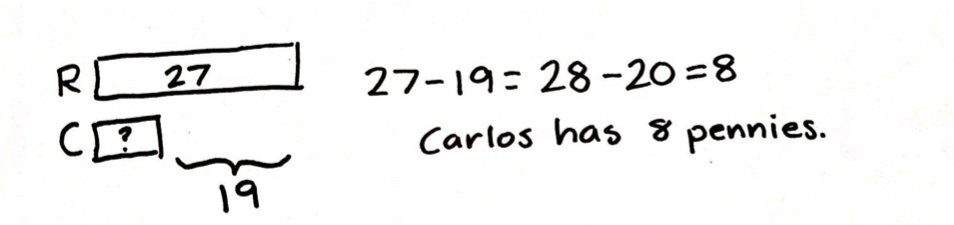Note: In this problem, the context shifts to money. This leads into today’s Concept Development, where students work with money data to solve word problems, and segues into problem solving with coins and bills in Topic B. The problem type is compare with smaller unknown, one of the more difficult problem types because more suggests the wrong operation. Guide students, as needed, to draw a tape diagram to solve.

HideShow

In the solution for this problem, the student uses a related problem to find the number of pennies Carlos has. The student adds 1 to 27 and adds 1 to 19; the difference of the related problem is the same.

## Two-step word problems

In Grade 2, students engage with two-step word problems for all subtypes, but because they’re still developing proficiency with the most challenging subtypes, it might be best to initially avoid these types in two-step problems. Also, most of the two-step problems students engage with should involve single-digit addends.7 ibid. p. 18. ibid. p. 18. ibid. p. 18. Since two-step problems are new to Grade 2, using smaller numbers allows students to focus on problem-solving rather than computation. Below are some variations of two-step problems.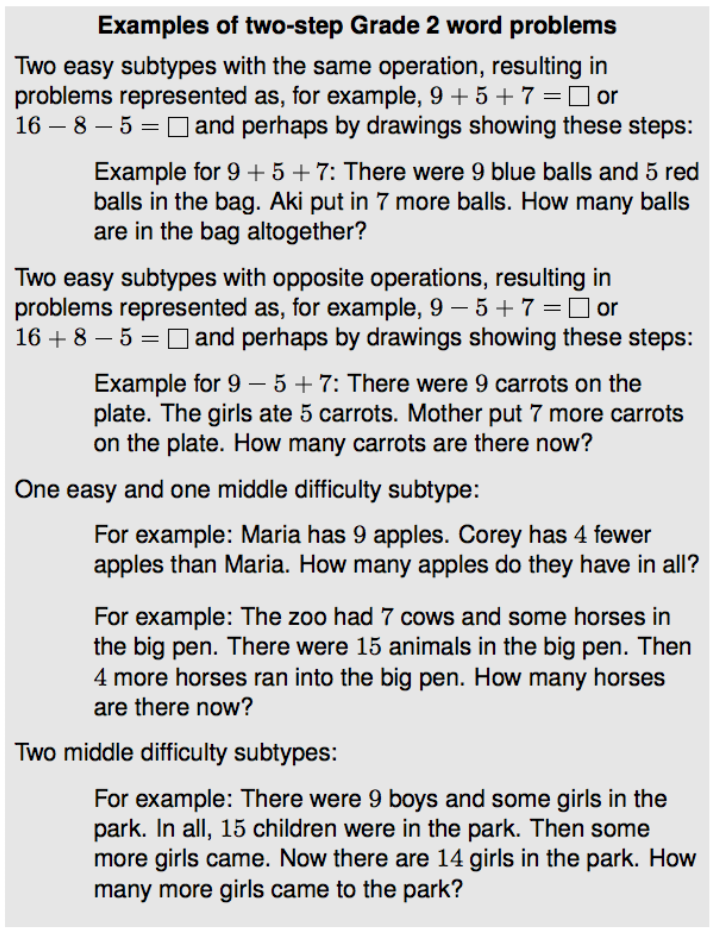Here’s another example, with sample solution:

#### A Pencil and a sticker

A pencil costs 59 cents, and a sticker costs 20 cents less. How much do a pencil and a sticker cost together?

Solution

The pencil costs 59 cents, and the sticker costs 20 cents less than that: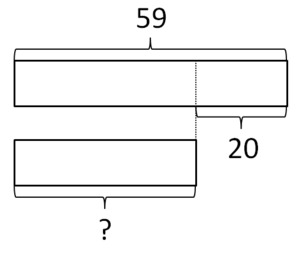So the sticker costs 59-20 = 39 cents.

The cost of the two together: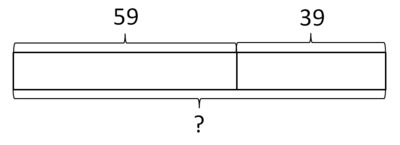is 59 + 39 = 98 cents.

HideShow

You’ll see that the numbers in this task are both two-digit. Though students do some work adding and subtracting with multiples of 10 in Grade 1, as in the first part, the second part involves Grade 2 skills and understandings.

When solving two-step problems like this one, we can support students with scaffolded questions to help them create representative drawings:

• “If this section represents the cost of a pencil, how could we represent the cost of a sticker?”
• “If this section represents the cost of a pencil, how could we represent the cost of a pencil and a sticker?”

Students use models (drawings and equations with a symbol for the unknown) to represent the problem situations described in Table 1. This helps them continue to develop meaning for addition and subtraction. As shown in in the two-step example above, tape diagrams are a good tool for compare problems. Tape diagrams can also be used for additive relationships; they just look a little different (See both Start Unknown examples.). Number bonds are also good tools for additive relationships.8 ibid. p. 16. ibid. p. 16. ibid. p. 16.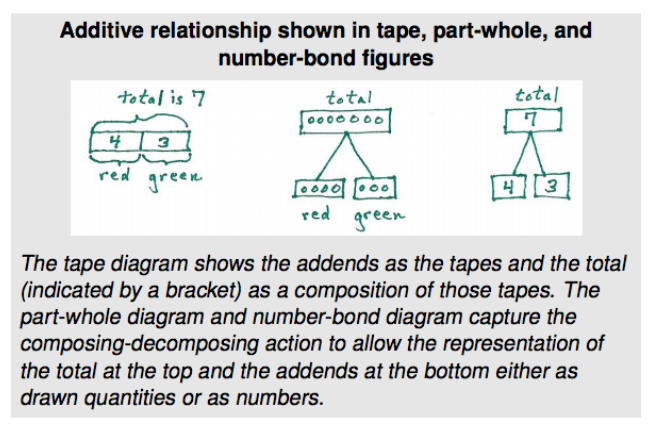As Grade 2 students become more advanced mathematicians, they begin replacing pictures of quantities with numbers in their diagrams (i.e., they move from drawing 7 circles to writing the number 7). They gradually increase their use of solution equations (i.e., the equation used to solve the problem) as representations over situation equations (i.e., the equation that represents the problem situation), and relate solution equations to diagrams.9 ibid. p. 17. ibid. p. 17. ibid. p. 17. For example, in the “add to start unknown example from EngageNY shown above about Susan and her piggy bank (EngageNY Grade 2, Module 4, Lesson 2: Application), the student represents the situation as an unknown addend problem (e.g., ? + 30 = 57). However, the student finds the solution by subtracting: 57 – 30 = 27. Facility with writing related addition and subtraction equations supports this work. Similarly, understanding that the total can be on the right or left side of the equal sign is an important student experience; for example, students should see that ? + 30 = 57 and 57 = ? + 30 are equivalent ways of representing the situation above. Having the total on the left helps students build on the understanding introduced in Grade 1 that the equal sign does not always mean “makes” or “results in” but always means “is the same number as”.10 ibid. p. 9. ibid. p. 9. ibid. p. 9.

# Part 2: How does problem solving with addition and subtraction relate to other parts of Grade 2?

There are lots of connections among standards in Grade 2; if you think about the standards long enough, you’ll probably start to see these relationships everywhere. In this section, we’ll talk about the connection between the Operations & Algebraic Thinking standards (from clusters 2.OA.A and 2.OA.B) and the standards in the fourth cluster of the Measurement & Data (MD) domain. The standards in this MD cluster are supporting standards and can be used to support work with the OA standards.

2.MD.D | Represent and interpret data.

 2.MD.D.9 Generate measurement data by measuring lengths of several objects to the nearest whole unit, or by making repeated measurements of the same object. Show the measurements by making a line plot, where the horizontal scale is marked off in whole-number units. 2.MD.D.10 Draw a picture graph and a bar graph (with single-unit scale) to represent a data set with up to four categories. Solve simple put-together, take-apart, and compare problems* using information presented in a bar graph. *See Glossary, Table 1

Data contexts are good places to reinforce problem-solving with addition and subtraction. In particular, they’re well-suited for supporting the compare subtypes. Students should have experiences generating measurement data, recording the data on a line plot, and then problem-solving with the data. In creating a line plot, students relate the horizontal axis to a number line. Number line diagrams are important tools for addition and subtraction in Grade 2 and rely on understandings from other MD clusters. (2.MD.A, 2.MD.B) In the following example, let’s see how students generate measurement data, create a line plot, and make observations about the data. Note the questions about comparing data.

#### Grade 2, Module 7, Lesson 24: Problem Set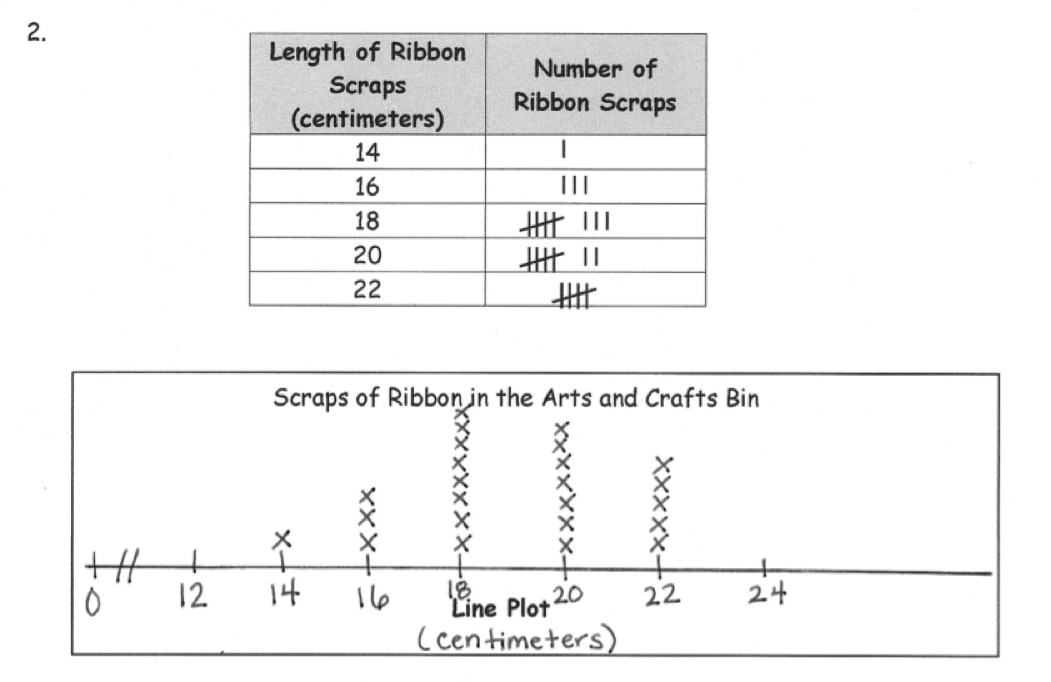a. Describe the pattern you see in the line plot.

b. How many ribbons are 18 centimeters or longer?

c. How many ribbons are 16 centimeters or shorter?

d. Create your own comparison question related to the data.

HideShow

Picture graphs and bar graphs are other opportunities to reinforce problem-solving. In fact, the language of the standard suggests as much: Solve simple put-together, take-apart, and compare problems using information presented in a bar graph. (2.MD.D.10) This standard even references Table 1. Though part of the standard addresses organizing and representing data, the focus of the standard is on interpreting data for the purposes of solving addition and subtraction problems. In the following example, students are asked to solve a simple compare problem.

#### Grade 2, Module 7, Lesson 4: Application

Materials: (T) Favorite animals bar graph (Template)

After a trip to the zoo, Ms. Anderson’s students voted on their favorite animals. Use the bar graph to answer the following questions.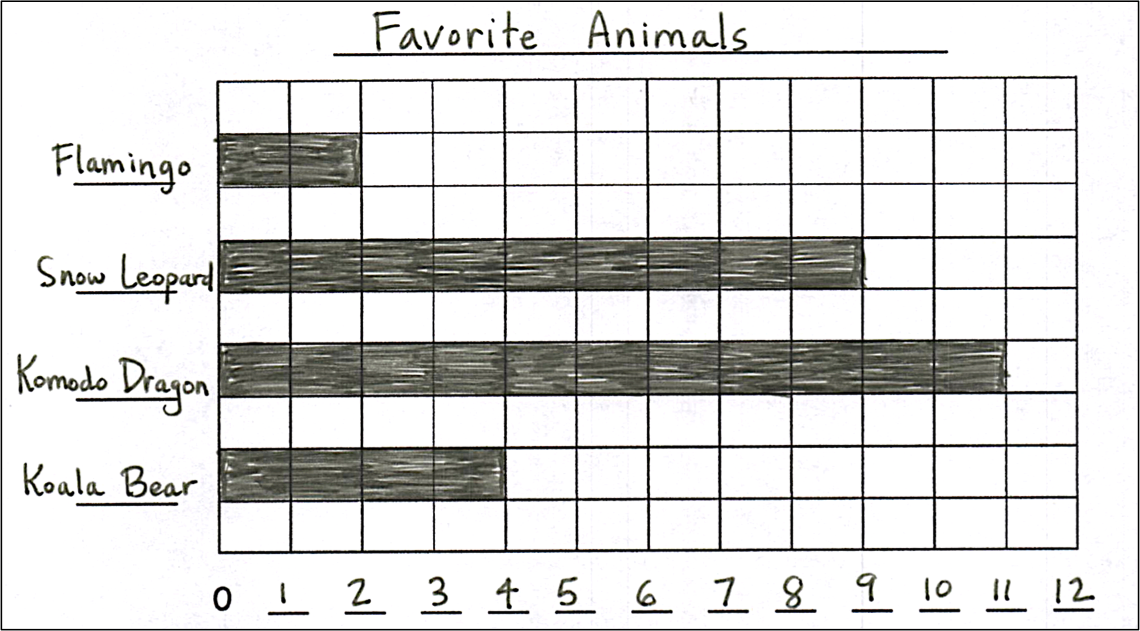1. Which animal got the fewest votes?
2. Which animal got the most votes?
3. How many more students liked komodo dragons than koala bears?
4. Later, two students changed their votes from koala bear to snow leopard. What was the difference between koala bears and snow leopards then?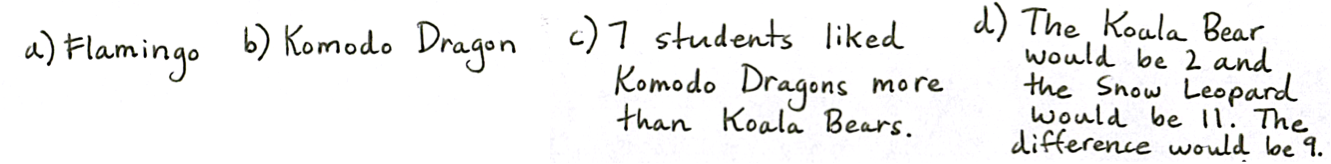Note: Project or draw this graph on the board. This problem reviews yesterday’s Concept Development, where students learned to read and interpret a bar graph. It leads into today’s lesson, where students create bar graphs to represent new data sets.

HideShow

Another meaningful across-grade connection is to the second cluster of the MD domain: Relate addition and subtraction to length. (2.MD.B) Experience with measuring and length provide access to measuring contexts for the different types of word problems; it also provides a conceptual basis for understanding the operations of addition and subtraction. In the example below, students engage with add to and compare situations with centimeters.

#### High Jump Competition

Presley jumped 36 cm on her first jump in the high jump competition. On her second jump, she jumped 45 cm.

1. How many total cm did Presley jump?

1. How many fewer cm did Presley jump on her first jump than her second jump?

1. Logan also jumped twice. The total of Logan's two jumps was 95 cm. How many more total cm did Logan jump than Presley?

# Part 3: Where do Operations & Algebraic Thinking come from, and where are they going?

As you’ve no doubt surmised, the big story in K-2 is about addition and subtraction. Standards from both the OA and the Counting & Cardinality (CC) domains are significant in defining these skills and understandings. Let’s take a closer look at this progression, specifically considering the strategies students learn, the fluencies they develop and problems they solve in each grade. This will allow us to think about how to explicitly connect new learning to previous understanding (and toward future learning), and about how to support students who may lack some prerequisite understandings.

Podcast clip: Importance of Coherence with Andrew Chen and Peter Coe (start 9:34, end 26:19)

As we’ve discussed, the Progressions describe three levels of problem representation and solution. Let’s see how the Standards show a progression of these strategies across these three grades.

 Kindergarten: “Counting all” within 10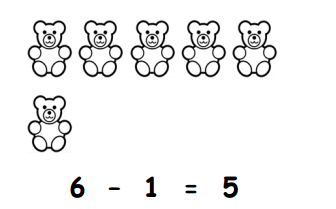➔ In Kindergarten, students connect counting to cardinality (i.e., the number of objects in a group)11 For students coming to second grade without the prerequisite understandings of the count sequence and of cardinality, please see our Content Guide on Counting & Cardinality in Kindergarten. For students coming to second grade without the prerequisite understandings of the count sequence and of cardinality, please see our Content Guide on Counting & Cardinality in Kindergarten. For students coming to second grade without the prerequisite understandings of the count sequence and of cardinality, please see our Content Guide on Counting & Cardinality in Kindergarten. and count to answer “How many?” questions. (K.CC.B.5) In keeping with the emphasis on counting, students use the Level 1 strategy of “counting all” to add and subtract, often using drawings or physical objects. (K.OA.A.2) In this example, students are directed to cross out bears to represent the problem. Students would “count all” of the remaining bears to determine that there are 5.

 Grade 1: Introducing “Counting on” within 20 T: While we were cleaning up, some of the beans fell on the carpet. I picked most of them up, but I think I am still missing some. We had 7 beans in total, right? S: Right! T: Now, I have 5 beans. (Show beans to the class.) T: How many am I missing? Talk with your partner to solve this. S: (Discuss.) T: Let’s try to count on to check how many I’m missing. S/T: Fivvvve (gesture to beans in hand), 6, 7. (Track on fingers.) T: How many did we count on to get up to 7? (Keep fingers out to show the two that were used to track.) S: Two! T: So, how many beans am I missing? S: Two beans! ➔ In Grade 1, students learn to “count on” to solve addition and subtraction problems, in addition to Level 3 strategies. (1.OA.C.6) In this lesson plan, the teacher works with students to count on to subtract 7 - 5; doing this also requires understanding subtraction as an unknown-addend problem (1.OA.B.4) (solving 7 - 5 = ? is the same as solving 5 + ? = 7).

 Grade 2: Mental strategies T: (Write 9 + 4 on the board.) T: Let’s draw to solve 9 + 4 using circles and Xs. T: (Quickly draw and count aloud 9 circles in a 5-group column as seen in the first image.)T: How many Xs will we add? S: 4 Xs. T: (Using the X symbol, complete the ten and draw the other 3 Xs to the right as seen in the second image.) T: Did we make a ten? S: Yes! T: Our 9 + 4 is now a ten-plus fact. What fact can you see in the drawing? S: 10 + 3 = 13. T: 10 + 3 equals? S: 13. T: So, 9 + 4 equals? S: 13. (Write the solution.) ➔ Students work to develop fluency with addition and subtraction within 20 in Grade 2. Students mentally apply the strategies developed in Grade 1 and know single-digit addition and subtraction facts from memory. (2.OA.C.3) Incorporating strategies based on place value (2.NBT.B.5) allows students to add and subtract larger numbers.

From a conceptual bases of counting and strategies, students develop fluency with addition and subtraction across these three grades. The expected fluency in Kindergarten is to add and subtract within 5. (K.OA.A.5) In Grade 1, the fluency expectation for adding and subtracting is within 10, (1.OA.C.6) and in Grade 2, the fluency expectation is for adding single-digit numbers within 20 (and the associated subtractions). (2.OA.B.2) As with all fluencies, the expectation is that students are fluent by the end of the year. Also, fluency results from extensive work making meaning of addition and subtraction as opposed to rote memorization. The following are examples of fluency practice exercises from these grades.

 Kindergarten: Addition and subtraction within 5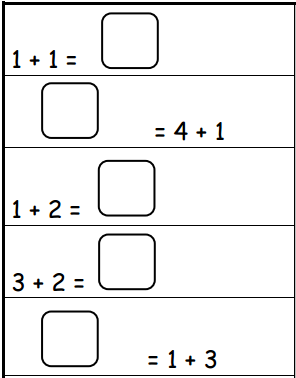➔ Students work to develop fluency with addition and subtraction within 5 in Kindergarten. (K.OA.A.5) Note the varied placement of the missing sums, which supports flexible thinking about the equal sign.

 Grade 1: Addition and subtraction within 10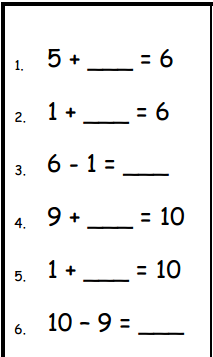➔ Students work to develop fluency with addition and subtraction within 10 in Grade 1. Note the proximity of pairs like 5 + ____ = 6 and 1 + ____ = 6, which highlights the commutative property. (1.OA.B.3) The proximity of pairs like 1 + ____ = 6 and 6 - 1 = ____ highlights subtraction as an unknown addend problem (1.OA.B.4) and the relationship between addition and subtraction. (1.OA.C.6)

 Grade 2: Addition and subtraction within 20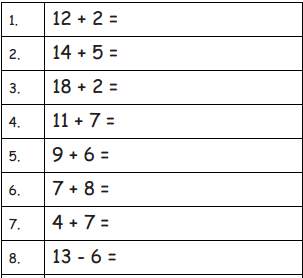➔ Students work to develop fluency with addition and subtraction within 20 in Grade 2. Students mentally apply the strategies developed in Grade 1 and know single-digit addition and subtraction facts from memory. (2.OA.C.3)

The adapted table from the standards, as shown in Part 1 of this guide, outlines the progression of addition and subtraction word problems across Grades K-2. Let’s take a closer look at these.

 Kindergarten: Four simple subtypes within 10 Julia went to the beach and found 3 seashells. Her sister Megan found 2 seashells. Draw the seashells the girls found. How many did they find in all? Talk to your partner about how you know. ➔ In Kindergarten, students solve “add to” and “take from” problems with result unknown and “put together/take apart” problems with total unknown and both addends unknown within 10. This is a “put together” problem (Julia’s shells and Megan’s shells are put together) with the total unknown (we want to know how many there are in all).

 Grade 2: All subtypes mastered within 100 Mei’s frog leaped several centimeters. Then, it leaped 34 centimeters. In all, it leaped 50 centimeters. How far did Mei’s frog leap at first? Draw a picture and write a number sentence to explain your thinking. ➔ In Grade 2, students master all subtypes, with an emphasis on work with the most challenging types: “add to” and “take from” problems again, this time with the start unknown; and certain more challenging compare problems. Students also solve two-step problems. This is an “add to with start unknown” problem (34 centimeters are added to several unknown centimeters to get 50 centimeters). Students need to figure out the number of centimeters the frog leaped initially.

### Suggestions for students who are below grade level

• If a significant number of students don’t have fluency with the count sequence, you could ensure daily choral practice as a “warm up” or other activity during the day. (This activity, which includes several counting activities, might be helpful for this purpose.)
• If a significant number of students don’t have a firm foundation with the Level 1 strategy of “counting all,” or the Level 2 strategy of “counting on,” you could plan a couple of lessons on these strategies prior to lessons that focus on Level 3 strategies. (This lesson, along with others in EngageNY’s Kindergarten Module 4, offers opportunities for students to add by counting concrete objects. This lesson, along with others in EngageNY’s Grade 1 Module 1, Topic D, offers opportunities for students to “count on.” To deliberately connect to Grade 2 standards, you might use the same examples to model or discuss other strategies, or apply the same ideas to larger numbers.)
• For students who lack fluency with addition and subtraction within 5 or 10, consider incorporating drills on these facts into your weekly routine. Strategy work, as named above, will be vital for fluency as well. Starting within 5 can then build naturally over time to fluency with larger numbers. (For an example of this type of activity, see the Core Fluency Practice in this lesson (Kindergarten) or this lesson (Grade 1).)

## Beyond K-2

Developing a solid understanding of addition and subtraction problem types in K-2 is important for developing understanding of multiplication and division. Students draw on their understanding of properties of addition, the relationship between addition and subtraction, and decomposition of numbers to build meaning for multiplication and division. Also, strong facility with addition and subtraction and related problem situations helps students to better distinguish multiplication and division from addition and subtraction. In the Grade 4 example below, students solve a two-step word problem involving addition and multiplication of whole numbers. By this point, students use the standard algorithm for multi-digit addition and subtraction and properties of operations (e.g., the distributive property, which is based on decomposing numbers) and area models to multiply.

#### EngageNY Grade 4, Module 3, Lesson 11: Problem Set

1. A restaurant sells 1,725 pounds of spaghetti and 925 pounds of linguini every month. After 9 months, how many pounds of pasta does the restaurant sell?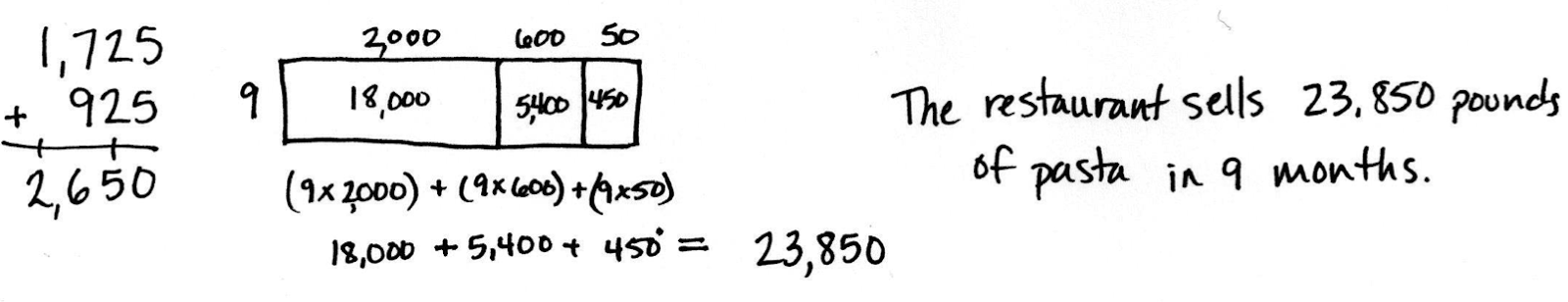Students need a firm understanding of which operations to apply and how to perform them. This comes from having many experiences developing meaning for the operations and using strategies rooted in conceptual understanding.

Though the standards in the OA domain concentrate on experience with whole numbers, the concepts, properties, methods, and representations extend beyond to other number systems. Students extend their engagement with the 12 subtypes to include addition and subtraction of fractions and decimals in Grades 3-5.12 ibid. p. 19. ibid. p. 19. ibid. p. 19. Ultimately students will bring a host of problem-solving strategies to bear when solving problems involving all four operations and the full scope of the rational number system.7.NS.B.3

Congratulations on making it through this Grade 2 guide for Operations & Algebraic Thinking with Connections to Data. We hope it provided a clear and detailed explanation of what these standards say, and how Operations & Algebraic Thinking relates to other mathematical concepts in Grade 2, as well as Kindergarten and Grade 1.

The content here can be helpful when writing or evaluating a scope and sequence, a unit plan or lesson plan. And you can find additional resources
here:

Student Achievement Partners: Focus in Grade 2

Draft K-5 Progression on Counting and Cardinality and Operations and Algebraic Thinking

EngageNY: Grade 2 Module 1 Materials

EngageNY: Grade 2 Module 4 Materials

# Endnotes

 The Common Core State Standards for Mathematics (CCSSM) are organized into major, additional, and supporting clusters in the Focus by Grade Level documents from Student Achievement Partners.

 For a more thorough discussion of these strategies you can consult our Grade 1 guide to Operations & Algebraic Thinking.

 ibid. p. 9.

 ibid. p. 18.

 ibid. p. 16.

 ibid. p. 17.

 ibid. p. 9.

 For students coming to second grade without the prerequisite understandings of the count sequence and of cardinality, please see our Content Guide on Counting & Cardinality in Kindergarten.

 ibid. p. 19.

# FAQs

## 1. What is a Content Guide?

Our goal in creating the Content Guides has been to provide busy teachers with a practical and easy-to-read resource on what the grade-level math standards are saying, along with examples of instructional materials that support conceptual understanding, problem-solving, and procedural skill and fluency for students.

It’s important to note that content guides are not meant to serve as a curriculum (or any kind of student-facing document), a guide or source material for test-preparation activities, or any kind of teacher evaluation tool.

## 2. What’s in a Content Guide?

Each Content Guide is focused on a specific group of standards. Most Content Guides follow the same three-part structure:

• Part 1 makes clear the student skills and understandings described by this group of standards. This section illustrates the standards using multiple student tasks from freely available online sources. Teachers can use or adapt these tasks for their students.
• Part 2 explains how this group of standards is connected to other standards in the same grade. We highlight how these connections have implications for planning and teaching, and how this within-grade coherence can increase access for students. Part 2 also includes multiple student tasks from freely available online sources.

• Part 3 traces selected progressions of learning leading to grade-level content discussed in the specific Content Guide. This discussion segues into a series of concrete and practical suggestions for how teachers can leverage the progressions to teach students who may not be prepared for grade-level mathematics. Finally, Part 3 traces the progression to content in higher grades.

## 3. How can I use the Content Guides?

Teachers who have read our Content Guides say they see benefits for all educators. Here are some suggestions for how different educators might use them.

Teachers can use the Mathematics Content Guides to:

• Increase or refresh their knowledge of the standards and the expectations for what students should know by the end of the year.
• Adapt lessons and units using appropriate pre-requisites to support students who are behind grade-level.
• Gain access to the best available OER for math to use for introducing and/or reinforcing concepts
• Ensure their curriculum and/or units:
• Focus on the major work of the grade and the appropriate depth of each standard.
• Target the appropriate aspects of rigor—procedural skill and fluency, modeling and application, and conceptual understanding described by the standards.
• Help students make coherent connections within and across grades.
• Create or revise their lessons and questioning to focus on important concepts in the standards.

Instructional coaches and school leaders can use the Mathematics Content Guides to:

• Refresh or increase their knowledge of the standards and the expectations for what students should know by the end of the year.
• Develop and communicate consistent expectations for lesson planning and instruction aligned to the standards.
• Provide a reference when planning and/or discussing instruction with teachers.
• Gain insight into what instruction and student work should look like in order to meet the demands of the standards.
• Develop and design content and standards-driven professional development sessions/workshops.
• Foster content rich, standards-based discussions among staff and build staff knowledge.
• Develop and/or revise school improvement plans in order to support and incorporate content and practice-based teaching and learning.

## 4. Why the Content Guides?

The transition to higher standards has led teachers all over the country to make significant changes in their planning and instruction, but only one-third of teachers feel they are prepared to help their students pass the more rigorous standards-aligned assessments (Kane et.al., 2016). This is to be expected because the new high standards are a significant departure from prior standards. The standards require a deeper level of understanding of the math content they teach; a different progression of what students need to learn by which grade; as well as different pedagogy that emphasizes student conceptual understanding, problem solving and procedural fluency in equal intensity.

The support for teachers to bring high standards to their classrooms, however, has lagged behind. Research shows that teacher training in the U.S. is currently insufficient in preparing teachers to teach the demanding new standards (Center for Research in Mathematics and Science Education, 2010). And though some resources exist that “unpack” the standards, few, if any, explain and illustrate the standards. “Unpacking” the standards one by one can also result in a disjointed presentation that neglects the structure and coherence of the standards. In creating the Content Guides, we aimed to provide busy teachers with a practical, easy-to-read resource on their grade-specific standards and how to help all students learn them. There is ample empirical evidence that when teachers have both strong knowledge of the math content that they teach, and the pedagogical knowledge to help students master that content knowledge, their students learn more (Baumert et. al., 2010; Hill, Rowan and Ball, 2005; Rockoff et. al., 2008). With the Content Guides in hand, we hope that teachers will find more success in helping their students make progress toward college- and career-readiness.

## 5. What is the relationship between the Content Guides and the Progressions?

The Progressions documents describe the grade-to-grade development of understanding of mathematics. These were informed by research on children’s cognitive development as well as the logical structure of mathematics. The Progressions explain why standards are sequenced the way they are. The Content Guides often highlight key ideas from the Progressions, but do not add new standards or change the expectations of what students should know and be able to do; they aim to explain and illustrate a group of standards at a time using freely available online sources. While the OER tasks and lessons in the Content Guides are one way to meet the grade-level standards, they are not the only means for doing so.

## 6. How were the resources selected?

We selected sample tasks and lessons from freely available online sources such as EngageNY, Illustrative Mathematics and Student Achievement Partners to illustrate the Standards. These sources are chosen because they are fully aligned to the new high standards based on national review of K-12 curricula or are created by organizations led by the writers of the new high standards. In addition, because they are open educational resources (OER), they are freely accessible for all uses. All UnboundEd materials are also OER, as part of our commitment to make high-quality, highly aligned content available to all educators.